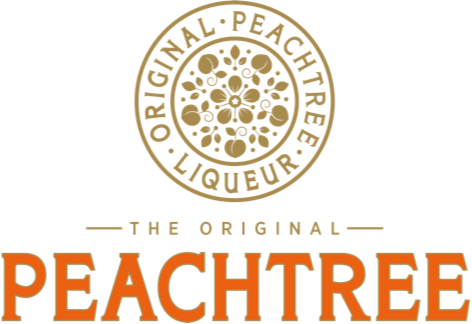# Nutritional value and allergens

Peachtree bottle (70cl, 1L) nutritional information

• No allergens
• All ingredients in Peachtree are suitable for vegans.
• Nutritional values per serve (40ml):
• Energy (kcal) = 91
• Energy (kJ) = 380
• Total fat (g) = 0
• Of which is saturated fat (g) = 0
• Carbohydrates (g) = 11,6
• Of which sugars (g) = 11,6
• Protein (g) = 0
• Sodium (g) = <0,01
• Salt (g) = <0,01

Fizzy Peachtree can (250ml) nutritional information

• No allergens
• All ingredients in Peachtree are suitable for vegans.
• Nutritional values per serve (250ml):
• Energy (kcal) = 150
• Energy (kJ) = 613
• Total fat (g) = 0
• Of which is saturated fat (g) = 0
• Carbohydrates (g) = 21,3
• Of which sugars (g) = 21,3
• Protein (g) = 0
• Sodium (g) = <0,01
• Salt (g) = <0,01